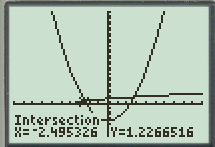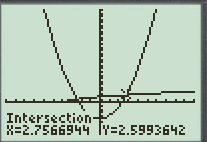# The solution of the equation x + 4 = x 2 − 5 graphically.### Precalculus: Mathematics for Calcu...

6th Edition
Stewart + 5 others
Publisher: Cengage Learning
ISBN: 9780840068071### Precalculus: Mathematics for Calcu...

6th Edition
Stewart + 5 others
Publisher: Cengage Learning
ISBN: 9780840068071

#### Solutions

Chapter 1, Problem 96RE
To determine

## To calculate: The solution of the equation x+4=x2−5 graphically.

Expert Solution

The solution of the equation x+4=x25 are x=2.5 and x=2.7 .

### Explanation of Solution

Given information:

The equation is x+4=x25

Calculation:

Consider the equation x+4=x25 .

Divide the above equation in two parts.

Follow the steps provided below to estimate the solution of the equation,

Step 1: Press Y= key, enter the function Y1=X+4 .

Step 2: Enter the function Y2=X25 .

Step 3: Press the TRACE key.

Step 4: Press 2nd key and then TRACE key.

Step 5: Choose the 5th option that is the intersect feature.

Step 6: Press ENTER key to select both the functions.

Step 7: Guess the intersect point and press ENTER key.

The result obtained on screen is provided below,The graphs intersect at (2.5,1.2) .

Step 8: Press 2nd key and then TRACE key.

Step 9: Choose the 5th option that is the intersect feature.

Step 10: Press ENTER key to select both the functions.

Step 11: Guess the intersect point and press ENTER key.

The result obtained on screen is provided below,The graphs intersect at (2.7,2.5) .

There are two points of intersection that depict the solution of the equation.

Thus, the solution of the equation x+4=x25 are x=2.5 and x=2.7 .

### Have a homework question?

Subscribe to bartleby learn! Ask subject matter experts 30 homework questions each month. Plus, you’ll have access to millions of step-by-step textbook answers!